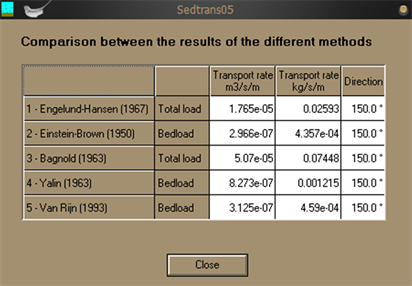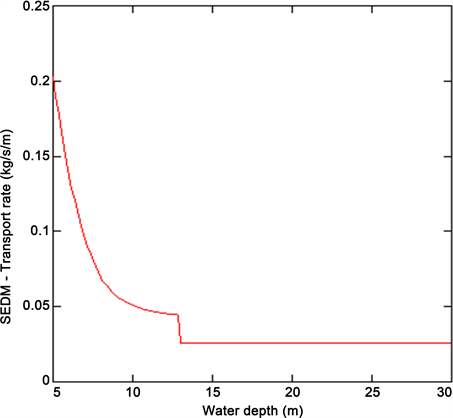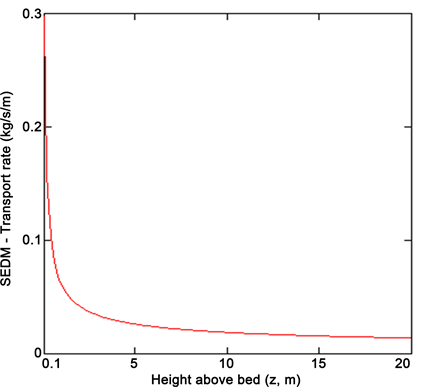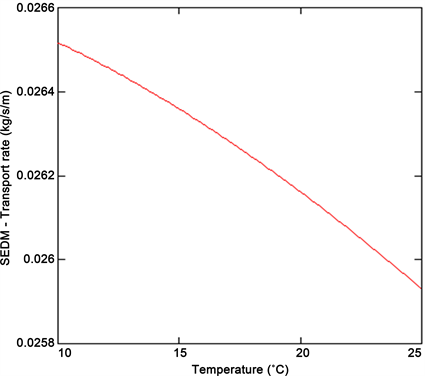# 基于sedtran05模型的悬浮物输送数值模拟方法A Numerical Simulation Method of Sediment Transport Based on sedtran05 Model

DOI: 10.12677/CSA.2019.95106, PDF, HTML, XML, 下载: 384  浏览: 1,203

Abstract: In order to implement the transport of sediment, one-dimensional numerical simulation method based on sedtran05 is proposed. Firstly, considering the density and viscosity of water, the settling velocity, and the transfer equation, the calculate method of vertical profile model is built. Secondly, the Yellow Sea environmental conditions simulation experiments are carried out based on sedtran05 model, calculating the sediment transport rate for different particle of sediment, depth, speed, temperature of seawater. Finally, by comparing the data with other literature with the simulation results, we verify the validity of method and define the scope of application of the model.

1. 引言

2. 垂直剖面输沙模型

Sedtrans模型由Nova Scotia于20世纪80年代提出  ，能够用于模拟稳定流或在海浪、海流综合影响下，沙子或粘性悬浮物的输运问题  。

2.1. 水的密度和粘度

$\begin{array}{l}\eta =1.802863×{10}^{-3}-6.1086×{10}^{-5}T+1.31419×{10}^{-6}{T}^{2}-1.35576×{10}^{-8}{T}^{3}\\ \text{\hspace{0.17em}}\text{\hspace{0.17em}}\text{\hspace{0.17em}}\text{\hspace{0.17em}}\text{\hspace{0.17em}}+2.15123×{10}^{-6}S+3.59406×{10}^{-11}{S}^{2}\end{array}$ (1)

2.2. 沉降流速

${W}_{s}=\frac{v}{D}\left[{\left({10.36}^{2}+1.049{D}_{*}^{3}\right)}^{0.5}-10.36\right]$ (2)

2.3. 启动再悬浮的临界剪切速度

$1<{D}_{*}\le 10:\frac{{u}_{*crs}}{{W}_{s}}=\frac{4}{{D}_{*}}$ (3)

2.4. 传输方程

$q=\alpha {\left(s-1\right)}^{0.5}{g}^{0.5}{D}^{1.5}{D}_{*}^{-0.3}{T}_{m}^{2.1}$ (4)

$q=0.25\alpha D{D}_{*}^{-0.3}{\left(\frac{{\tau }_{cws}}{\rho }\right)}^{0.5}{T}_{m}^{1.5}$ (5)

3. 仿真实验

3.1. 泥沙粒径的影响(0.01~0.075 mm)Figure 1. Result of conveying sand with different output equation in sedtran05Figure 2. The relationship between the transport rate and sediment particle size

3.2. 水深的影响(5~30 m)

3.3. 水流流速的影响(0.1~1 m/s)Figure 3. The relationship between the transport rate and depth of waterFigure 4. The relationship between the transport rate and velocity

3.4. 海床上方高度的影响(0.1~20 m)Figure 5. The relationship between the transport rate and sea level

3.5. 温度的影响(10℃~25℃)Figure 6. The relationship between the transport rate and temperature

4. 结语

*通讯作者。

  孙湘平. 近海区域海洋学[M]. 北京: 海洋出版社, 2006: 95-248.  Qiu, Z.F., Xiao, C., Perrie, W., et al. (2017) Using Landsat 8 Data to Estimate Suspended Particulate Matter in the Yellow River Estuary. Journal of Geophysical Research: Oceans, 122, 276-290. https://doi.org/10.1002/2016JC012412  尹小青, 杨顶田, 周立柱. 黄、东海边界悬浮物输运量的卫星遥感估算[J]. 热带海洋学报, 2018, 37(2): 10-16.  Cai, L.N., Tang, D.L., Levy, G., et al. (2016) Remote Sensing of the Impacts of Construction in Coastal Waters on Suspended Particulate Matter con Centration—The Case of the Yangtze River Delta, China. International Journal of Remote Sensing, 37, 2132-2147. https://doi.org/10.1080/01431161.2015.1121302  Cai, L.N., Tang, D.L. and Li, C.Y. (2015) An Investigation of Spatial Variation of Suspended Sediment Concentration Induced by a Bay Bridge Based on Landsat TM and OLI Data. Advances in Space Research, 56, 293-303. https://doi.org/10.1016/j.asr.2015.04.015  Yang, X.F., Mao, Z.H., Huang, H.Q., et al. (2016) Using GOCI Retrieval Data to Initialize and Validate a Sediment Transport Model for Monitoring Diurnal Variation of SSC in Hangzhou Bay, China. Water, 8, 108-113. https://doi.org/10.3390/w8030108  陆海建. 二维风浪潮流泥沙数学模型在天津港新建滚装码头工程中的应用[D]: [硕士学位论文]. 天津: 天津大学, 2010.  孙文心, 江文胜, 李磊. 近海环境流体动力学数值模型[M]. 北京: 科学出版社, 2004: 333-356.  宋永港. 海南岛重点区沙滩波流输沙模型[D]: [硕士学位论文]. 上海: 华东师范大学, 2011.  王飞, 王珊珊, 王新, 等. 杭州湾悬浮泥沙遥感反演与变化动力分析[J]. 华中师范大学学报(自然科学版), 2014, 48(1): 112-116, 135.